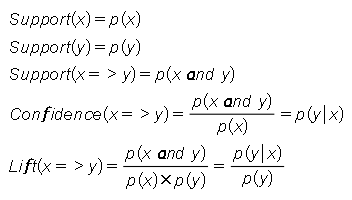Support(x)p(x)

Support(y)p(y)

Support(x=>y)p(x and y)

Confidence(x=>y)=  f(x and y)/f(x) = p(x and y)/p(x)p(y|x)

Lift(x=>y)p(x and y)/p(x)×p(y)p(y|x)p(y)p(x and y)<= p(x)

p(x and y)<= p(y)

p(x and y)<     p(x and y)/p(x)

p(x and y)<     p(x and y)/p(y)

p(x and y)<     p(x and y)/(p(x)p(y))

 Topic Access A Gentle Introduction on Market Basket Analysis — Association Rules https://towardsdatascience.com/a-gentle-introduction-on-market-basket-analysis-association-rules-fa4b986a40ce Complete guide to Association Rules (1/2) https://towardsdatascience.com/association-rules-2-aa9a77241654 Complete guide to Association Rules (1/2) https://www.kdnuggets.com/2016/04/association-rules-apriori-algorithm-tutorial.html Association rule learning https://en.wikipedia.org/wiki/Association_rule_learning#cite_note-measurescomp-10 Market basket analysis for Business Analytics (20 min) https://www.youtube.com/watch?v=jAPDu9pR5aw Association Rules or Market Basket Analysis (10 min) https://www.youtube.com/watch?v=91CmrpD-4Fw&

#### Articles

 A Gentle Introduction on Market Basket Analysis — Association Rules Exercises in R https://towardsdatascience.com/a-gentle-introduction-on-market-basket-analysis-association-rules-fa4b986a40ce

Since 11 April 2023: 215 total views,  1 views today